# SQLite String Functions: REPLACE, SUBSTR, TRIM, ROUND (Examples)

[vc_row][vc_column][vc_column_text]SQLite equipped by default, with a list of built-in functions within the SQLite library. You can also add some other custom functions using the C language to extend the core functions.

In this tutorial, you will learn-

Note that, for all the following examples, you have to run the sqlite3.exe and open a connection to the sample database as flowing:

Step 1) In this step,

1. Open My Computer and navigate to the following directory “C:\sqlite” and then
2. open “sqlite3.exe“:Step 2) Open the database “TutorialsSampleDB.db” by the following command:Now you are ready to run any query and try any queries used in the following sections.

## Finding the LENGTH of a string

To find the length of a string use the LENGTH(X) where X is a string value. If X is a null value, the length function will return a null value.

You can also use the length function with numeric values to get the length of the numeric value.

Example

In the following example, we will try the LENGTH function syntax with values as following:

`SELECT LENGTH('A string'), LENGTH(NULL), LENGTH(20), LENGTH(20.5);`

This will give you:The result is as following:

• LENGTH(‘A string’) returns 8 which is the length of the string “A string”.
• LENGTH(NULL) returns null.
• LENGTH(20) returns 2, because 20 is 2 lengths long.
• LENGTH(20.5) returns 4; The floating point “.” is counted as a character, so you will have four characters – the 3 characters which are the length of the numbers. Plus one more character for the floating point “.”.

## Changing case with UPPER Function and LOWER Function

UPPER(X) will return the same x string, but it will represent all the string characters in capital characters.

LOWER(X) will return the same x string, but it will represent all the string characters in small characters.

UPPER and LOWER returns null values if you pass a null value to them.

If you pass numeric values to UPPER or LOWER, both of them will return the exact numeric value.

Example

`SELECT UPPER('a string'), LOWER('A STRING'), UPPER(20), LOWER(20), UPPER(NULL), LOWER(NULL);`

This will give you: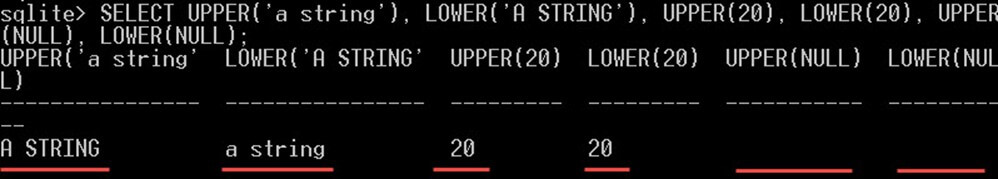The result is as following:

• UPPER(‘a string’) returns the string “a string” in capital letter representation “A STRING“.
• LOWER(‘A STRING’) returns the string “A STRING” in small letter representation “a string“.
• UPPER(20), LOWER(20) returns the same number, as they don’t have any effect on numbers.
• UPPER(NULL), LOWER(NULL) returns null because we passed a null value to them.

## SQLite SUBSTR Function

SUBSTR function returns a specific number of string, starting from a specific position. You can pass three operands to the function, like this “SUBSTR(X,Y,Z)” as following:

• X is the string literal or the string column to parse. You can pass a literal value (static value) or a column name, and in this case, the value will be read from the column’s values.
• Y is the starting position to subtract the string starting from it.
• Z is the number of characters to parse starting from the specific position Y. This number is optional you can ignore it, and in this case, SQLite will subtract the string starting from the position Y tell the end of the string.

Example

In the following query, we will use the SUBSTR function to get the 4 characters starting from the second character from the students’ names:

```SELECT StudentName, SUBSTR(StudentName, 2, 4), SUBSTR(StudentName, 2)
FROM Students;```

This will give you: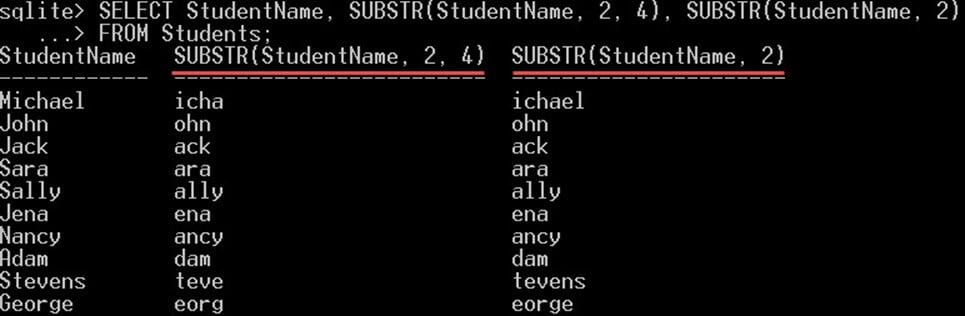• SUBSTR(StudentName, 2, 4) substring the string “StudentName” starting from the second character, and return the next 4 characters.
• However, in the function SUBSTR(StudentName, 2) we didn’t specify a number for the characters to be returned, that’s why SQLite returned all the remaining characters in the “StudentName” starting from the second position.

## Changing parts of a string with SQLite REPLACE

REPLACE is used to replace each string occurred on another string with a string.

REPLACE(X, Y, Z) – X is the input string literal or column. Y is the string to replace with the string Z. Note that it will replace any occurrence of the string Y with Z, not just one.

Example

In the following query, we will replace all the occurrence of the string “xx” with the string “SQLite“:

`SELECT REPLACE('xx is very lightweight, xx is easy to learn', 'xx', 'SQLite');`

This will give you• The replace function replaced all the strings “xx” with the string “SQLite“.
• So the output will be “SQLite is very lightweight, SQLite is easy to learn”.

## Trimming blank spaces with TRIM Function

TRIM remove blank spaces from the beginning or from the end of the string. It won’t remove any spaces in the middle of the string, only from the beginning or from the end.

Example:

In the following query, we will use the TRIM function to remove the blank spaces from the beginning and the end of the string.

Note that, the concatenation operator “||” is used to add an extra “;” to the end of the string. To show you that the empty spaces were removed in the black window.

`SELECT TRIM('      SQLite is easy to learn        ') || ';';`

This will give you: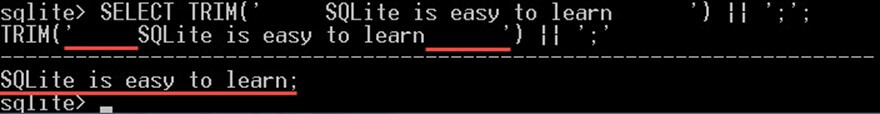• Notice how the blank spaces was removed from the start and from the end of the string, replaced by ‘ ; ‘ at the end.

## Reading absolute values with ABS Function

The ABS function returns the absolute value of a numeric value. The absolute value is the numeric value without any positive or negative sign. ABS(X) returns a value depending on the value of X as following:

• The absolute value of X if X is a numeric value.
• A NULL value if X is a null value.
• “0.0” value if X is a string.

Example

In the following query, we will try to get the absolute value of a string, a number, and a null value using the ABS function:

`SELECT ABS(-2), ABS(+2), ABS('a string'), ABS(null);`

This will give you: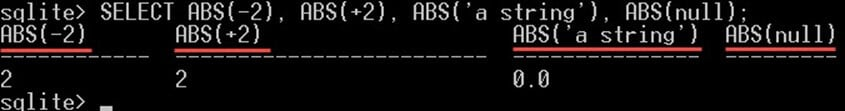The results as following:

• ABS(-2), ABS(+2) returns 2, because 2 is absolute value for both “-2” and “+2”.
• ABS(‘a string’) returns “0.0” because you passed a string value not a numeric value.
• ABS(null) returns null because you passed a null value for it.

## Rounding values with ROUND Function

Decimal numbers are numbers with floating point “.” on it, for example, “20.5”, “8.65”. The part on the left of the floating point is called the integer part, and the part on the right of the floating point is the decimal part.

Integer numbers are those numbers without any floating point on it. For example, “20”, “8”.

ROUND(X) converts the decimal values or columns X to be integer numbers only. The integer part which is the part on the left of the decimal point will be returned, and all the digits to the right of the decimal point will be removed.

Example:

In the following query we will try the ROUND function with different options:

`SELECT ROUND(12.4354354), ROUND(12.4354354, 2), ROUND(NULL), ROUND('a string');`

This will give you: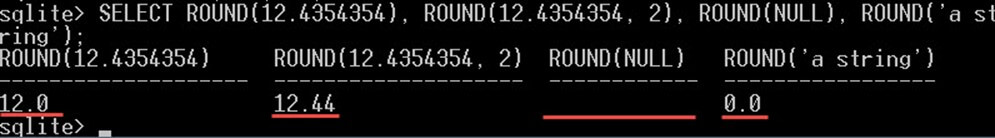The result as following:

• ROUND(12.4354354) – returns the value “12”, because you didn’t specify the number of digits. So, SQLite removed all the decimal part.
• ROUND(12.4354354, 2) – returns the value “12.44”, because you specified only 2 digits to round which are the two digits from the right of the decimal point.
• ROUND(NULL) – returns null because you passed a null value to it.
• ROUND(‘a string’) – returns the value “0.0” because you passed a string value to it.

## Finding the data type of expression with TYPEOF Function

If you want to find out the type of a column or a literal value, you can use the function TYPEOF to do that.

The TYPEOF(X) function, from its name, returns the data type of the expression X. It returns a string value indicating the data type of X. It returns the value based on the datatypes, whether it is a “NULL” value, real, text, integer, …, etc.

Example

In the following query we will try the TYPEOF function with different types of literal values:

`SELECT TYPEOF(null), TYPEOF(12), TYPEOF(12.5), TYPEOF('a string');`

This will give you:The results returned is as following:

• TYPEOF(null) – returns null because you passed a null value to it.
• TYPEOF(12) – return an integer as 12 is an integer number.
• TYPEOF(12.5) – returns REAL as 12.5 is a real number.
• TYPEOF(‘a string’) – returns text as “a string” is a text.

## Finding the last inserted record with SQLite LAST_INSERT_ROWID

SQLite assigns an integer key (Id) for all the rows in all the tables. This number is used to uniquely identify these rows.

When you INSERT a new row on a table, SQLite will assign the rowid a unique value.

If the table has a primary key declared on one column only and that column is of data type INTEGER, then this column value will be used as rowid.

The LAST_INSERT_ROWID() function returns the ROW_ID of the last inserted row in any table in the database. Note that it doesn’t take any operand.

Example

In the following example we will run the function LAST_INSERT_ROWID() as follows:

`SELECT LAST_INSERT_ROWID();`

This will give you:• LAST_INSERT_ROWID() return 0 because there are no rows inserted into any tables in the current database connection.

Now, let’s insert a new student and run the function LAST_INSERT_ROWID() again after the insert statement, like this:

```INSERT INTO Students VALUES(11, 'guru', 1, '1998-10-12');
SELECT LAST_INSERT_ROWID();```

This will give you: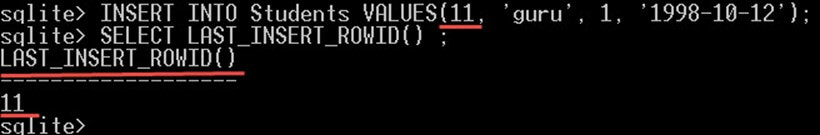• After we inserted a new student with id 11, the LAST_INSERT_ROWID() will return the last inserted row id which is 11.

## Getting the version of your SQLite library

To get the version of your SQLite library, you have to call the SQLITE_VERSION() function.

Example

Let’s find out what is the version of the SQLite we are using by running the following command:

`SELECT SQLITE_VERSION();`

This will give you: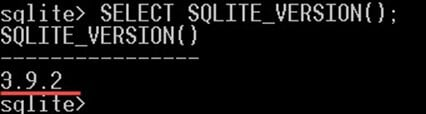SQLITE_VERSION() returns 3.9.2 which is the version of the SQLite we are using.

## Creating user-defined functions and aggregate user-defined functions

For some users, the SQLite core functions are limited. They might need to add custom functions for their custom needs.

SQLite doesn’t support creating user-defined functions. Unlike other database management systems, you can’t create user defined functions in SQLite directly.

However, you can create a function using programming languages like C#, C, PHP, or C++ and attached that function with the core SQLite functions in the SQLite library itself, using “sqlite3_create_function” function. Then you can reuse them in your database.

Example:

In the following example we will create a user defined function using C# programming language and add it to SQLite functions:

```[SQLiteFunction(Name = "DoubleValue", Arguments = 1, FuncType = FunctionType.Scalar)]
public class DoubleValue: SQLiteFunction
{
public override object Invoke(object[] args)
{
return args * 2;
}
}```

This code snippet is written in the C# programming language; it creates a C# function using the C# code.

The function name will be “DoubleValue” it takes one parameter and returns its value multiplied by 2.

Note that C# automatically add this function to SQLite. All that you need is to compile and run this code. Then C# will add that function with the same name to the list of SQLite functions. Then you can use this function in SQLite.

The same way using programming languages like C#, C, PHP, or C++, you can also create aggregate user-defined functions. Which are used to extend the aggregate functions in SQLite and use them to create custom aggregate functions.

Summary

SQLite provides a comprehensive set of built-in functions that make working with database’s tables and columns easy as we saw. You can use these functions with columns as well as literal values within any expression in your SQL queries.[/vc_column_text][/vc_column][/vc_row]